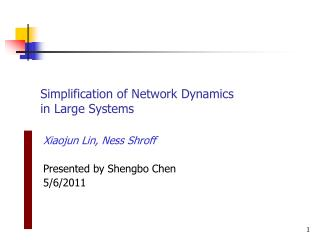DownloadDownload PresentationSimplification of Network Dynamics in Large Systems

# Simplification of Network Dynamics in Large Systems

Download Presentation## Simplification of Network Dynamics in Large Systems

- - - - - - - - - - - - - - - - - - - - - - - - - - - E N D - - - - - - - - - - - - - - - - - - - - - - - - - - -
##### Presentation Transcript

1. Simplification of Network Dynamicsin Large Systems Xiaojun Lin, Ness Shroff Presented by Shengbo Chen 5/6/2011

2. Outlines • Introduction • System model • Problem statement • Main result • Conclusion

3. Introduction • Large Networks • Internet • Power network • Network Pricing • Dynamic pricing: charge different prices to the users according to the current utilization level of the network and also other factors • Better performance • Static pricing: price is independent of the current network utilization • Do not require instantaneous load information • Only depends on average parameters • Less overhead and computation

4. System model • Network with L links • Link capacity • I users • Price • Poisson arrivals with rate • Resource cost • Feasible States where is the number of flows, and is the routing matrix

5. System model (contd’) • Service time • Dynamic pricing function where is an increasing sequence of positive numbers

6. Problem Statement • Revenue maximization where pik is the probability that the service time Ti is in the segment [ak-1,ak)

7. Upper and Lower Bounds • Upper bound • Lower bound

8. Scaling Laws • Scaling Laws 1 • Scaling factor c: increasing sequence to infinity • Scaling Laws 2 • Scaling with topology changes Number of links, number of classes, routing matrix

9. Main Result • Under the scaling law 1 • Under the scaling law 2 • Dynamic routing does not help The static prices induced by the upper bound are in fact asymptotically optimal The static prices induced by the upper bound is not asymptotically optimal

10. Intuition & Implication • The upper bound has the average constraint, which is the loosest. • Strong Law of Large Number • Sands in a can • Implications • An appropriately chosen static scheme can approach that of the optimal dynamic scheme when the system is large • Telephone networks • Smart grid

11. Future work • Delay tolerant task?

12. Conclusion • An appropriately chosen static scheme can approach that of the optimal dynamic scheme when the system is large • Static pricing scheme: lack of adaptivity • Dynamic pricing scheme: different from the duality-based scheme